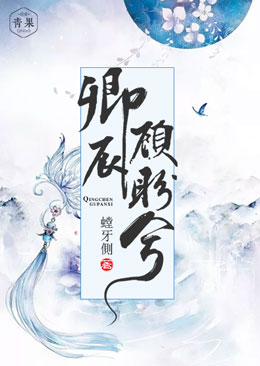## 目录### 卿辰顾盼兮作者：安呤• 楔子
• 朱雀
• 行刑台上的少年
• 下凡
• 再相遇
• 神兽
• 进宫
• 我是陆卿辰
• 莫名情愫
• 华妃召见
• 初见舞榭
• 四皇子陆卿茗
• 治理水患
• 同行
• 进城
• 小乞丐
• 帮忙
• 出手
• 知县公子
• 男女通吃
• 初入府中
• 见到知县夫人
• 史瑜的怪异
• 知县召见
• 入书房
• 知县的逼问
• 史瑜夫子
• 出发江北
• 史瑜见到顾盼
• 冤家
• 路遇山贼
• 收编山贼
• 荣城
• 丧尸
• 丧尸围城
• 顾盼显形
• 妖族太子元烈
• 骷髅花
• 开战
• 主动出击
• 连城
• 再见故人
• 往事
• 妖族要反
• 魔怪
• 斗魔怪
• 出手相救
• 苏醒
• 被丧尸包围
• 进入城主府
• 终于见到你
• 调查水患
• 妖龙作怪
• 妖龙出水
• 交手
• 入魔
• 消灭妖龙
• 顾盼重伤
• 顾盼苏醒
• 女娲的宠爱
• 陆卿辰的心思
• 水患的根源
• 落脚
• 进山
• 降妖除魔
• 陷入噬灵阵法
• 阵眼是活的
• 自己送上门的小团子
• 小团子是雌性么
• 破阵
• 跟随
• 继续出发
• 梦魇
• 魔尊骨遥
• 诛仙
• 前世今生
• 顾盼没死
• 现实与梦境
• 总裁苏祜
• 不一样的总裁
• 暧昧
• 打脸了
• 康复出院
• 到底哪个才是梦
• 不要离开我
• 顾盼疯了
• 婚礼
• 禁忌之恋
• 不真实的温柔
• 下棋
• 史瑜进宫
• 陆卿辰被赐婚
• 当场拒婚
• 贵妃拦路
• 被迫成亲
• 两个人的婚礼
• 新过门的皇子妃
• 与皇子妃杠上
• 向贵妃娘娘哭诉
• 向贵妃娘娘告状
• 竹苑暴露
• 贵妃生气
• 上官怜儿被罚
• 倒霉的上官怜儿
• 竹苑的人
• 恋情败露
• 谋划计策
• 上官怜儿的奸情
• 上官怜儿回府
• 紧急诏令
• 相赴黄泉
• 梦醒
• 魔族
• 骨倩
• 是哥哥吗
• 风雨欲来
• 顾盼受伤
• 玄冰长老
• 收徒
• 找茬
• 救人
• 采薇
• 田大爷是妖怪
• 麻烦来了
• 六尾白狐
• 田大爷是女的
• 被放逐的狐狸
• 七巧身世
• 跟我走
• 错把珍珠当鱼目
• 出发百断山脉
• 秘法
• 灼烧秘法
• 成功破解
• 修罗场###### 行刑台上的少年
2018-01-03 08:14 更新

# 行刑台上的少年

“真的？什么时候？”

“就刚刚，现在正在行刑台呢，估摸着时间，应该很快就要被贬下凡了吧。”

“不过话说，如果他肯归顺我们天族的话，倒是众仙子的倾慕之人……”

“不跑了？”白虎开口道。

“不去啊？也行，我最多就是反咬你一口，说是你让我去的。我就是替你去看看情况而已。”说完就自顾自的往前面走去。

###### (本章完)## App下载